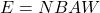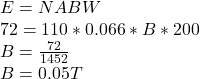## A rectangular coil 20 cm by 33 cm has 110 turns. This coil produces a maximum emf of 72 V when it rotates with an angular speed of 200 rad/s

Question

A rectangular coil 20 cm by 33 cm has 110 turns. This coil produces a maximum emf of 72 V when it rotates with an angular speed of 200 rad/s in a magnetic field of strength B.Find the value of B.

in progress 0
5 months 2021-09-04T11:48:17+00:00 2 Answers 2 views 0

1. Explanation:

Below is an attachment containing the solution.

0.05T

Explanation:

Data given,

area, A=20cm*33cm=0.2m*0.33m=0.066m^2

Number of turns, N=110 turns,

Emf= 72v,

magnetic field strength, B= ??

from the expression showing the relationship between induced emf and magnetic field is shown belowWhere N is the number of turns,

E=is the emf,

Bis the magnetic field strength

if we substitute values, we arrive at